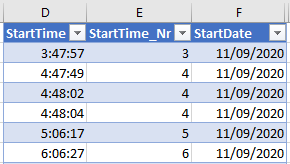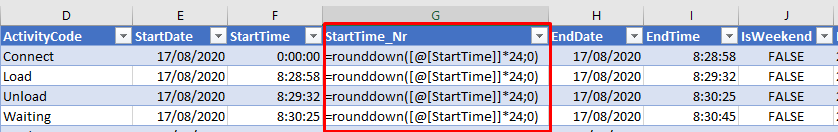Hello,

The following NP formula - that needs to report the hour of a specific StartTime as a number from 0  to 24 - gives the correct result in a small report with only 3 columns:

=@NP("Formula";"=rounddown([@[StartTime]]*24;0)")

Results see column EHowever, when adding more columns in the report (10 columns in total) the formula is not calculated anymore. The formula is displayed as a text:Does anyone has an idea why this formula is no longer being calculated in a larger report?

Kind regards,

Inge